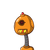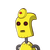# if length and breadth of the rectrangle is 2.5cm and 1.8 cm then find its area and perimeter​

if length and breadth of the rectrangle is 2.5cm and 1.8 cm then find its area and perimeter​

### 2 thoughts on “if length and breadth of the rectrangle is 2.5cm and 1.8 cm then find its area and perimeter​”

1.### ☯ GIVEN :

• Length of the rectangle : 2.5 cm
• Breadth of the rectangle : 1.8 cm

### ☯ TO FIND :

• Area and Perimeter.

### ☯ FORMULA REQUIRED :

$$\bigstar{\underline{\boxed{\red{\bf{{Area =Length\times Breadth }}}}}}$$

$$\bigstar{\underline{\boxed{\red{\bf{{Perimeter =2(Length+Breadth) }}}}}}$$

### ☯ SOLUTION :

$$\implies {\sf{Area =Length\times Breadth}} \\ \\$$

$$\implies {\sf{Area =2.5\times1.8 }} \\ \\$$

$$\implies { \underline{\boxed{ \red{\bf{Area =4.5 \: cm^2 }}}}}$$

$$\huge{\pink{\therefore}}$$ Area = $$\bf{4.5 \: cm^2}$$

### _________________________

$$\implies {\sf{Perimeter =2(Length + Breadth)}} \\ \\$$

$$\implies {\sf{ Perimeter=2(2.5 + 1.8) }} \\ \\$$

$$\implies {\sf{ Perimeter=2 \times 4.3 }} \\ \\$$

$$\implies { \underline{\boxed{ \pink{\bf{Perimeter =8.6 \: cm }}}}}$$

$$\huge{\red{\therefore}}$$ Perimeter = $$\bf{8.6 \: cm}$$

2.### Solution

GIVEN,

Length of the rectangle ⇛ 2.5

Breadth of the rectangle ⇛ 1.8

## To find

AREA AND PERIMETER

## Formula

1) Area = length X breadth

2) perimeter = 1 ( length + breadth)

### ─━━━━━━⊱✿⊰━━━━━━─

Solving

➣ Area = length X breadth

➣ Area = 2.5 X 1.8

➣ Area = 4.5 cm²

Area 4.5 cm²

_____________(✪)___________

Perimeter = 2 ( length + breadth)

➤ perimeter = 2( 2.5 + 1.8)

➤ perimeter = 2 ( 4.3)

➤ perimeter = 8.6cm

●❯────────────────❮●